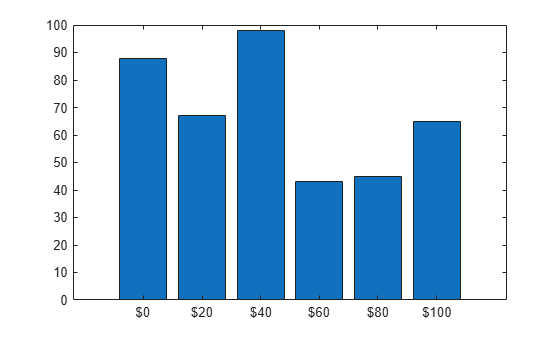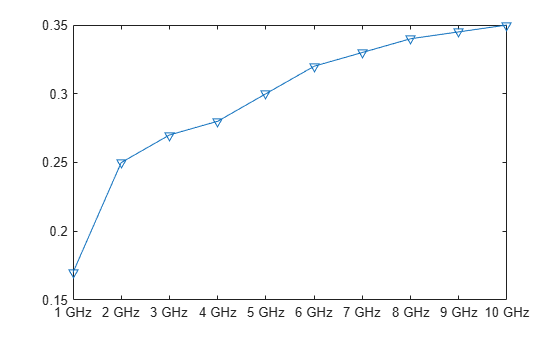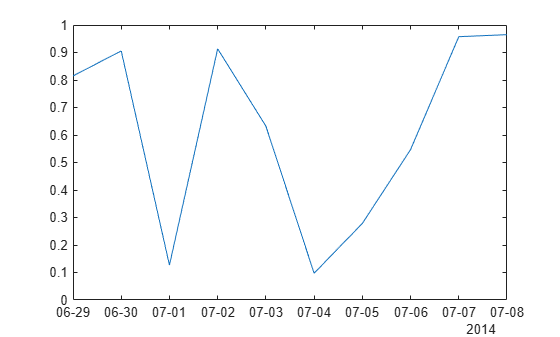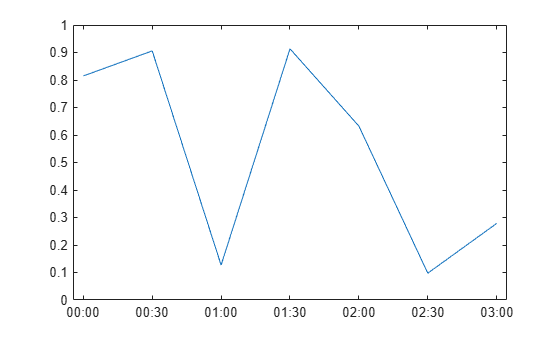# xtickformat

Specify x-axis tick label format

## Syntax

``xtickformat(fmt)``
``xtickformat(datefmt)``
``xtickformat(durationfmt)``
``xtickformat(ax,___)``
``xfmt = xtickformat``
``xfmt = xtickformat(ax)``

## Description

example

````xtickformat(fmt)` sets the format for numeric x-axis tick labels. For example, specify `fmt` as `'usd'` to display the labels in U.S. dollars.```

example

````xtickformat(datefmt)` sets the format for labels that show dates or times. For example, specify `datefmt` as `'MM-dd-yy'` to display dates such as `04-19-16`. This option applies only to an x-axis with datetime values.```

example

````xtickformat(durationfmt)` sets the format for labels that show durations. For example, specify `durationfmt` as `'m'` to display the durations in minutes. This option applies only to an x-axis with duration values.```

example

````xtickformat(ax,___)` uses the axes specified by `ax` instead of the current axes. Specify `ax` as the first input argument.```

example

````xfmt = xtickformat` returns the format style used for x-axis tick labels of the current axes. Depending on the type of labels along the x-axis, `xfmt` is a character vector of a numeric format, date format, or duration format.```
````xfmt = xtickformat(ax)` returns the format style used for the axes specified by `ax` instead of the current axes.```

## Examples

collapse all

Display x-axis tick labels in dollars with and without decimal values.

Create a bar chart. Display the tick labels along the x-axis in dollars.

```x = 0:20:100; y = [88 67 98 43 45 65]; bar(x,y) xtickformat('usd')```Query the tick label format. MATLAB® returns the format as a character vector containing the formatting operators.

`fmt = xtickformat`
```fmt = '\$%,.2f' ```

Display the tick labels with no decimal values by tweaking the numeric format to use a precision value of 0 instead of 2.

`xtickformat('\$%,.0f')`After creating a line chart, display the tick labels along the x-axis with the text "GHz" after each value.

```x = 1:10; y = [.17 .25 .27 .28 .3 .32 .33 .34 .345 .35]; plot(x,y,'-V') xtickformat('%g GHz')```After creating a scatter plot, display the x-axis tick labels with two decimal places. Control the decimal places by passing `xtickformat` a character vector of a numeric format that uses fixed-point notation for the conversion character and a precision value of 2.

```x = linspace(0,1,100); y = randn(1,100).*cos(x); scatter(x,y) xtickformat('%.2f')```Create a line plot with datetime values along the x-axis. Then, change the format of the dates.

```t = datetime(2014,6,28) + caldays(1:10); y = rand(1,10); plot(t,y); xtickformat('MM-dd')```Create a line plot with duration values along the x-axis. Then, change the format of the tick labels.

```t = 0:seconds(30):minutes(3); y = rand(1,7); plot(t,y) xtickformat('mm:ss')```Create a scatter plot and display the x-axis tick labels in Euro. Then, query the tick label format. MATLAB® returns the format as a character vector containing the formatting operators.

```x = rand(100,1); y = rand(100,1); scatter(x,y) xtickformat('eur')````xfmt = xtickformat`
```xfmt = '\x20AC%,.2f' ```

Starting in R2019b, you can display a tiling of plots using the `tiledlayout` and `nexttile` functions. Call the `tiledlayout` function to create a 2-by-1 tiled chart layout. Call the `nexttile` function to create the axes objects `ax1` and `ax2`. Plot into each of the axes. Specify the tick label format for the x-axis of the lower plot by specifying `ax2` as the first input argument to `xtickformat`. Display the tick labels in U.S. dollars.

```tiledlayout(2,1) ax1 = nexttile; plot(ax1,rand(6)) ax2 = nexttile; plot(ax2,rand(6)) xtickformat(ax2,'usd')```## Input Arguments

collapse all

Format for numeric tick labels, specified as a character vector or string scalar. You can specify one of the formats listed in this table. Alternatively, you can specify a custom format.

Predefined FormatDescription
`'usd'`

U.S. dollars. This option is equivalent using `'\$%,.2f'`. If the labels use scientific notation, this option sets the exponent value to 0.

`'eur'`

Euro. This option is equivalent to using `'\x20AC%,.2f'` with an exponent value of 0.

`'gbp'`

British pound. This option is equivalent to using `'\x00A3%,.2f'` with an exponent value of 0.

`'jpy'`

Japanese yen. This option is equivalent to using `'\x00A5%,d'` with an exponent value of 0.

`'degrees'`

Display degree symbol after values. This option is equivalent to using `'%g\x00B0'` with the default exponent value.

`'percentage'`

Display percent sign after values. This option is equivalent to using `'%g%%'` with the default exponent value.

`'auto'`

Default format of `'%g'` with the default exponent value.

Example: `xtickformat('usd')`

#### Custom Numeric Format

You can specify a custom numeric format by creating a character vector or string containing identifiers.Identifiers are optional, except the percent sign and conversion character. Construct the format in this order:

• One or more flags — Options such as adding a plus sign before positive values. For a full list of options, see the table of Optional Flags.

• Field width — Minimum number of characters to print in the tick label. Specify the field width as an integer value. If the number of significant digits in the tick value is smaller than the field width, then the label is padded with spaces.

• Precision — Number of digits to the right of the decimal point or the number of significant digits, depending on the conversion character. Specify the precision as an integer value.

• Conversion character — Value type. For a full list of options, see the table of Conversion Characters. If you specify a conversion that does not fit the data, then MATLAB® overrides the specified conversion, and uses `%e`.

Also, you can specify literal text at the beginning or end of the format. To print a single quotation mark, use `''`. To print a percent character, use `%%`.

Example: `xtickformat('%.2f')` displays the values using fixed-point notation with two decimal places.

Example: `xtickformat('\$%.2f')` displays a dollar sign before each value.

Example: `xtickformat('%.2f million')` displays `million` after each value.

Optional Flags

IdentifierDescriptionExample of Numeric Format
`,`Display commas every three digits, such as `'1,000'`.`'%,4.4g'`
`+`Print the sign character (+) for positive values, such as `'+100'`.`'%+4.4g'`
`0`Pad the field width with leading zeros instead of spaces, such as `'0100'`.`'%04.4g'`
`–`Left-justify, which pads the end of the value with spaces instead of the beginning. For example, if the field width is `4`, then this flag formats the label as `'100 '` instead of `' 100'`.`'%-4.4g'`
`#`

For the `%f`, `%e`, and `%g` conversion characters, print the decimal point even when the precision is 0, such as `'100.'`. For `%g`, do not remove trailing zeros.

`'%#4.4g'`

Conversion Characters

IdentifierDescriptionExample
`d` or `i`Signed integer with base 10. The precision value indicates the number of significant digits. `'%.4d'` displays π as `0003`.
`f`Fixed-point notation. The precision value indicates the number of decimal places.`'%.4f'` displays π as `3.1416`.
`e`Exponential notation. The precision value indicates the number of decimal places.`'%.4e'` displays π as `3.1416x100`.
`g`The more compact version of `e` or `f`, with no trailing zeros. The precision value indicates the maximum number of decimal places.`'%.4g'` displays π as `3.1416`.

Format for dates and times, specified as `'auto'`, a character vector, or a string scalar. The default format is based on the data.

Example: `xtickformat('yyyy-MM-dd')` displays a date and time such as `2016-04-19`.

Example: `xtickformat('eeee, MMMM d, yyyy HH:mm:ss')` displays a date and time such as `Saturday, April 19, 2016 21:41:06`.

Example: `xtickformat('MMMM d, yyyy HH:mm:ss Z')` displays a date and time such as `April 19, 2016 21:41:06 -0400`.

The display format tables show the letter identifiers that you can use to construct the format. To separate the fields, you can include nonletter characters such as a hyphen, space, colon, or any non-ASCII characters. The identifiers correspond to the Unicode® Locale Data Markup Language (LDML) standard for dates.

#### Date and Time Formats

Use these identifiers to specify the display formats of the date and time fields.

Letter IdentifierDescriptionDisplay
`G`Era`CE`
`y`Year, with no leading zeros. See the Note that follows this table.`2014`
`yy`Year, using last two digits. See the Note that follows this table.`14`
`yyy`, `yyyy` ...Year, using at least the number of digits specified by the number of instances of `'y'`For the year 2014, `'yyy'` displays `2014`, while `'yyyyy'` displays `02014`.
`u`, `uu`, ...ISO year. A single number designating the year. An ISO year value assigns positive values to CE years and negative values to BCE years, with 1 BCE being year 0.`2014`
`Q`Quarter, using one digit`2`
`QQ`Quarter, using two digits`02`
`QQQ`Quarter, abbreviated`Q2`
`QQQQ`Quarter, full name`2nd quarter`
`M`Month, numerical using one or two digits`4`
`MM`Month, numerical using two digits`04`
`MMM`Month, abbreviated name`Apr`
`MMMM`Month, full name`April`
`MMMMM`Month, capitalized first letter`A`
`W`Week of the month`1`
`d`Day of the month, using one or two digits`5`
`dd`Day of the month using two digits`05`
`D`Day of the year, using one, two or three digits`95`
`DD`Day of the year using two digits`95`
`DDD`Day of the year using three digits`095`
`e`Day of the week, numerical using one or two digits.`7`, where Sunday is the first day of the week.
`ee`Day of the week, numerical using two digits`07`
`eee`Day, abbreviated name`Sat`
`eeee`Day, full name`Saturday`
`eeeee`Day, capitalized first letter`S`
`a`Day period (AM or PM)`PM`
`h`Hour, 12-hour clock notation using one or two digits`9`
`hh`Hour, 12-hour clock notation using two digits`09`
`H`Hour, 24-hour clock notation using one or two digits`21`
`HH`Hour, 24-hour clock notation using two digits`21`
`m`Minute, using one or two digits`41`
`mm`Minute, using two digits`41`
`s`Second, using one or two digits`6`
`ss`Second, using two digits`06`
`S`, `SS`, ..., `SSSSSSSSS`Fractional second, using the number of digits specified by the number of instances of `'S'` (up to 9 digits).`'SSS'` truncates 6.12345 seconds to `123`.

Some tips and considerations:

• Use one or more `u` characters instead of `y` characters to represent the year when working with year numbers near zero.

• Datetime values later than 144683 years CE or before 140743 BCE display only the year numbers, regardless of the specified format value.

#### Time Zone Offset Formats

Use these identifiers to specify the display format of the time zone offset. A time zone offset is the amount of time that a specific date and time is offset from UTC. A time zone offset is different from a time zone in that it comprises rules that determine the offsets for specific times of the year. Include a time zone offset identifier when you want to ensure that the time components are displayed unambiguously.

Letter IdentifierDescriptionDisplay
`z`Abbreviated name of the time zone offset. If this value is not available, then the time zone offset uses the short UTC format, such as `UTC-4`.`EDT`
`Z`ISO 8601 basic format with hours, minutes, and optional seconds fields.`-0400`
`ZZZZ`Long UTC format.`UTC-04:00`
`ZZZZZ`ISO 8601 extended format with hours, minutes, and optional seconds fields. A time offset of zero is displayed as the ISO 8601 UTC indicator “Z”.`-04:00`
`x` or `X`ISO 8601 basic format with hours field and optional minutes field. If you specify `X`, a time offset of zero is displayed as the ISO 8601 UTC indicator “Z”.`-04`
`xx` or `XX`ISO 8601 basic format with hours and minutes fields. If you specify `XX`, a time offset of zero is displayed as the ISO 8601 UTC indicator “Z”.`-0400`
`xxx` or `XXX`ISO 8601 extended format with hours and minutes fields. If you specify `XXX`, a time offset of zero is displayed as the ISO 8601 UTC indicator “Z”.`-04:00`
`xxxx` or `XXXX`ISO 8601 basic format with hours, minutes, and optional seconds fields. If you specify `XXXX`, a time offset of zero is displayed as the ISO 8601 UTC indicator “Z”.`-0400`
`xxxxx` or `XXXXX`ISO 8601 extended format with hours, minutes, and optional seconds fields. If you specify `XXXXX`, a time offset of zero is displayed as the ISO 8601 UTC indicator “Z”.`-04:00`

Format for duration values, specified as a character vector or string scalar.

To display a duration as a single number that includes a fractional part (for example, 1.234 hours), specify one of the following formats.

Format Description
`'y'`Number of exact, fixed-length years. A fixed-length year is equal to 365.2425 days.
`'d'`Number of exact, fixed-length days. A fixed-length day is equal to 24 hours.
`'h'`Number of hours
`'m'`Number of minutes
`'s'`Number of seconds

To display a duration in the form of a digital timer, specify one of these formats:

• `'dd:hh:mm:ss'`

• `'hh:mm:ss'`

• `'mm:ss'`

• `'hh:mm'`

In addition, you can display up to nine fractional second digits by appending up to nine `S` characters. For example, `'hh:mm:ss.SSS'` displays the milliseconds of a duration value to three digits.

Target axes, specified as a single `Axes` object or a vector of `Axes` objects. If you do not specify the axes, then `xtickformat` uses the current axes.

## Algorithms

The `xtickformat` function sets and queries the `TickLabelFormat` property of the ruler object associated with the x-axis.